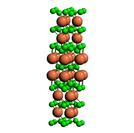Crystal Lattice Structures: Creation Date:  7 Feb 2003 Last Modified: 21 Oct 2004

# The Mo2B5 (D8i) Crystal StructureYou can now

• Compare this structure to W2B5 (D8h) . In both the Boron atoms form buckled graphite sheets.

• Prototype: Mo2B5
• Pearson Symbol: hR7
• Strukturbericht Designation: D8i
• Space Group: R3m (Cartesian and lattice coordinate listings available)
• Number: 166
• Reference: Wyckoff Vol II, pp. 189-90.
• Other compounds with this structure: V2B5, InL5Tl, Li5Sn2, Li5Tl2
• Primitive Vectors:  A1 = + ahex/2 X - ahex/(2 31/2) Y + chex/3 Z A2 = + ahex/31/2 Y + chex/3 Z A3 = - ahex/2 X - ahex/(2 31/2) Y + chex/3 Z
• Basis Vectors:  B1 = + ½(A1 + A2 + A3) = + ½chex Z (B-I) (2b) B2 = + z1(A1 + A2 + A3) = + z1chex Z (B-II) (2c) B3 = - z1(A1 + A2 + A3) = - z1chex Z (B-II) (2c) B4 = + z2(A1 + A2 + A3) = + z2chex Z (B-III) (2c) B5 = - z2(A1 + A2 + A3) = - z2chex Z (B-III) (2c) B6 = + z3(A1 + A2 + A3) = + z3chex Z (Mo) (2c) B7 = - z3(A1 + A2 + A3) = - z3chex Z (Mo) (2c)

Go back to the Carbon Structures page.

Go back to Crystal Lattice Structure page.

 Structures indexed by: This is a mirror of an old page created at theNaval Research LaboratoryCenter for Computational Materials ScienceThe maintained successor is hosted at http://www.aflowlib.org/CrystalDatabase/ and published as M. Mehl et al., Comput. Mater. Sci. 136 (Supp.), S1-S828 (2017).